The rules of signs for forces and moments

In the compilation of the equations of statics for systems in equilibrium (for example, in determining the support reactions), the rules of signs can be simplified to the following form:
Loads directed to one side are assumed to be positive, and accordingly loads of the opposite direction are written with a minus sign.

Signs of the projection of forces

That is, for the equations of sums of projections of forces on the axis:
Projections of forces and loads on the coordinate axis having the same direction are taken as positive, and projections of forces of the opposite direction are negative.

Video by subject (contains English subtitles)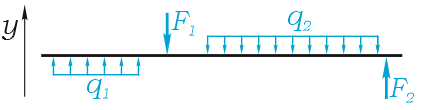the equation of the sum of forces has the form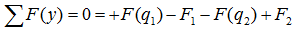And since the sums of the projections of the multidirectional forces are equal, then the given equation can also be written as: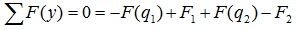Here, F (q) is the resultant of the distributed load, determined by the product of the intensity of the load on its length.

Video by subject (contains English subtitles)

Signs of Moments

The concentrated moments and moments of forces seeking to rotate the system with respect to the point under consideration along the clockwise direction are written into equations with one sign, and accordingly the moments having the opposite direction with the opposite sign.
For example, for the sum of the moments with respect to the point A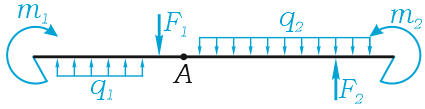we get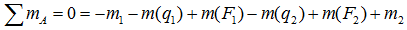or, that is the same thing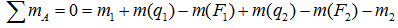Here m (F) are moments of forces F relative to point A.
M (q) are moments of distributed loads q with respect to the point under consideration.